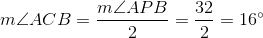## Example Questions

### Example Question #1 : How To Find The Percentage Of A Sector From An Angle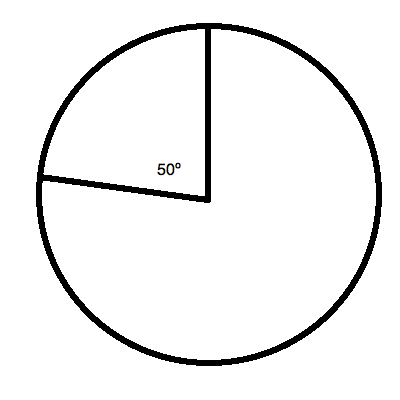The sector above represents what percentage of the complete circle? Round to the nearest hundredth.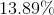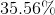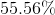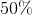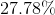Explanation:

This question is very easy to solve. You merely need to find what percentagedegrees is in comparison with the whole of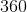degrees for the circle. This is: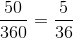or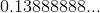or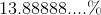Rounding, you get.

### Example Question #2 : How To Find The Percentage Of A Sector From An Angle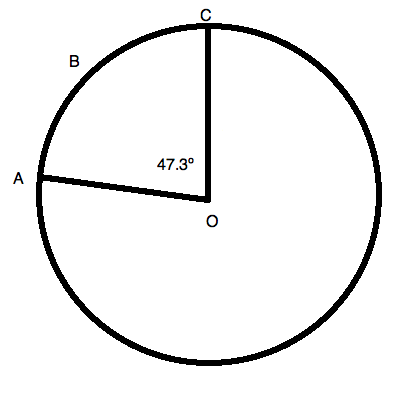Figure not drawn to scale.

What percentage of the circle is sector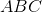? Round to the nearest hundredth of a percent.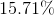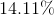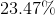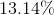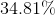Explanation:

Do not overthink this question! All you need to remember is that a given circle containsdegrees. This means that your sector percentage is merely a ratio of the angle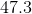to. This is: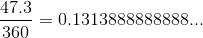or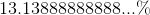Rounded, this is.

### Example Question #3 : How To Find The Percentage Of A Sector From An Angle

A sector of a circle is bounded by the minor arc of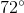. What percentage of the circle is the arc?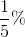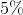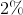Explanation:

The only information provided is that the sector is. When finding a percent, it's helpful to remember that percents are a clean way to show fractions—that is, portions—of a whole.

Therefore, in order to solve for what percent the sector represents of the entire circle, we need to find out what fraction the sector makes up of the entire circle. Keep in mind that a circle measures up to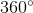. This means that the sector isof the total. This can be written out as: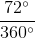This can be simplified to:Note that the degrees signs are gone. This is because when you divide degrees by degrees, the units cancel out! This simplified fraction means thatwas one-fifth of the entire circle. Now, this fraction may be turned into a percent.

In order to go from fractions to percents, it's helpful to keep in mind that decimals are the important intermediate step. That is, as soon as a fraction can be turned into decimal form, it can easily be converted into a percent by multiplying by 100.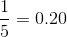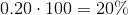That is, the sector makes upof the entire circle.

### Example Question #4 : How To Find The Percentage Of A Sector From An Angle

A sector of an angle is bounded the major arc of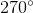. What percent of the circle does this make up?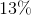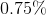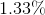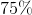Explanation:

The only information provided is that the sector is. When finding a percent, it's helpful to remember that percents are a clean way to show fractions—that is portions—of a whole. In order to solve for what percent the sector makes of the entire circle, we need to find out what fraction the sector makes up of the entire circle.

Keep in mind that a circle measuresdegrees. This means that the sector isof the total. This can be written out as: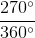This can be simplified to:Note that the degrees signs are gone. This is because when you divide degrees by degrees, the units cancel out! This simplified fraction means thatwas three-fourths of the entire circle. Now, this fraction may be turned into a percent.

In order to go from fractions to percents, it's helpful to keep in mind that decimals are the important intermediate step. That is, as soon as a fraction can be turned into decimal form, it can easily be converted into a percent by multiplying by.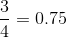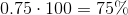That is, the sector makes upof the entire circle.

### Example Question #41 : Circles

In circle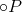, central angle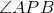and inscribed angle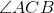both intercept minor arc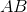. If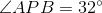, what is the measure of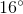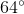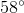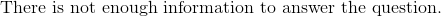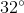A central angle and the arc it intercepts always have the same angle, and an inscribed angle always has an angle half the measure of the arc it intercepts. Thus, if the central angle measures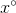, the corresponding inscribed angle must measure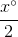.
Therefore,